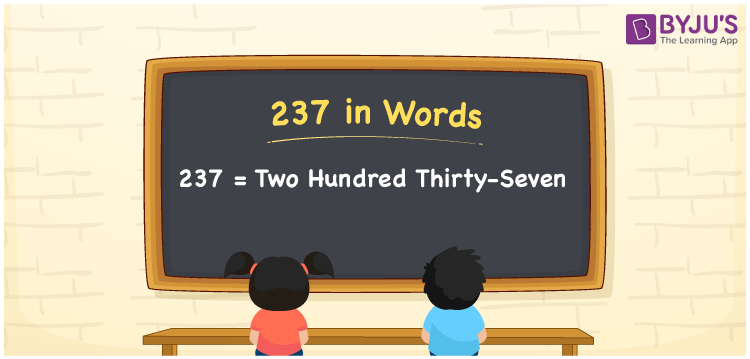# 237 in Words

In English words, the numeral 237 is expressed as “two hundred thirty-seven”. For example, if you bought a fantasy book worth Rs. 237, you can write it as “I bought a fantasy book worth Rs. Two hundred thirty-seven”. Also, the number 237 is a cardinal number, as well as a natural number.

 237 in Words: Two Hundred Thirty-seven. Two Hundred Thirty-seven in Numerical Form: 237.

## 237 in English Words## How to Write 237 in Words?

The place value table for the three-digit number 237 is given below.

 Hundreds Tens Ones 2 3 7

The expanded form of 237 is as follows:

= 2 × Hundred + 3 × Ten + 7 × One

= 2 × 100 + 3 × 10 + 7 × 1

= 200 + 30 + 7

= 237

= Two hundred thirty-seven

Hence, 237 in words is two hundred thirty-seven.

237 in words – Two hundred thirty-seven

Is 237 an odd number? – Yes

Is 237 an even number? – No

Is 237 a perfect square number? – No

Is 237 a perfect cube number? – No

Is 237 a prime number? – No

Is 237 a composite number? – Yes

## Frequently Asked Questions on 237 in Words

Q1

### How to spell 237 in words?

237 in words is two hundred thirty-seven.

Q2

### Simplify 200 + 37, and express it in words.

Simplifying 200 + 37, we get 237. Hence, 237 in words is two hundred thirty-seven.

Q3

### Is 237 a composite number?

Yes, 237 is a composite number.